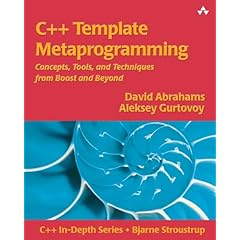# What I'm reading now: "C++ Template Metaprogramming"

I'm currently reading "C++ Template Metaprogramming". It's off to a great start.The basic idea (so far) is that:

1. You can write entire algorithms / programs in C++ templates, which are then executed as the compiler instantiates the template.

2. There is a discipline, structure, organization to these programs.

3. The template programs are generally recursive and heavily use template specialization for the base case.

Here's a simple example of writing Factorial like this.

``````
// Simple demo of writing Factorial via a template
#include "stdafx.h"

// Recursive case: Factorial(N) = Factorial(N-1) * N
template <int N>
struct Factorial
{
public:
static const int value = Factorial<N-1>::value * N;
};

// Use Template Specialization for base case: Factorial(1) = 1
template <>
struct Factorial<1>
{
static const int value = 1;
};

void _tmain(int argc, _TCHAR* argv[])
{
int v = Factorial<5>::value;

printf("%d\n", v); // prints 120
}
``````

In this case, Factorial<5> is completely computed at compile time.  Code generated for Factorial<5> is:

``````
int v = Factorial<5>::value;
004113AE  mov         dword ptr [v],78h  // 78h = 120 = 5 factorial

printf("%d\n", v); // prints 120
``````

The Boost libraries heavily make use of these techniques. And it will certainly be helpful in fully appreciating what happens in STL.